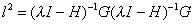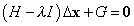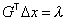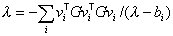ERRATA

Corrections to Reviews in Mineralogy Volume 42 ñ

Molecular Modeling Theory: Applications in the Geosciences

Chapter 14 "Molecular Orbital Modeling and Transition State Theory in Geochemistry "

pages 485-531

Mihali A. Felipe, Yitian Xiao, and James D. Kubicki

On page 503, with reference to Equations (54), (57) and (58) and the text preceding equation (59), the equations and text should read:(54)(57)(58)

If we express Equation (57) in terms of a diagonal Hessian representation, it rearranges to Equation (53). Substituting into Equation (58), we get(59)